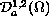USA-Chile Workshop on Nonlinear Analysis,
Electron. J. Diff. Eqns., Conf. 06, 2001, pp. 89-99.

### A one-dimensional nonlinear degenerate elliptic equation Florin Catrina & Zhi-Qiang Wang

Abstract:
We study the one-dimensional version of the Euler-Lagrange equation associated to finding the best constant in the Caffarelli-Kohn-Nirenberg inequalities. We give a complete description of all non-negative solutions which exist in a suitable weighted Sobolev space. Using these results we are able to extend the parameter range for the inequalities in higher dimensions when we consider radial functions only, and gain some useful information about the radial solutions in the N-dimensional case.

Published January 8, 2001.
Math Subject Classifications: 35J20, 35J70.
Key Words: best constant, ground state solutions, wighted Sobolev inequalities.

Show me the PDF file (145K), TEX file, and other files for this article.

Florin Catrina
Department of Mathematics and Statistics
Utah State University
Logan, UT 84322, USA.

Zhi-Qiang Wang
Department of Mathematics and Statistics
Utah State University
Logan, UT 84322, USA.
e-mail: wang@sunfs.math.usu.edu